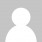# International Candidate Issues

• This forum has 143 topics, 574 replies, and was last updated 2 months ago byJenepher.
Viewing 30 topics - 1 through 30 (of 143 total)
Viewing 30 topics - 1 through 30 (of 143 total)
Create New Topic in “International Candidate Issues”
`<a class="" href="" title="" rel="" style="" download="" target=""> <abbr> <blockquote class="" style="" cite=""> <div class="" style=""> <span class="" style=""> <code class="" style=""> <pre class="" style=""> <em class="" style=""> <i class="" style=""> <b class="" style=""> <strong class="" style=""> <del datetime="" class="" style=""> <h1 align="" class="" style=""> <h2 align="" class="" style=""> <h3 align="" class="" style=""> <h4 align="" class="" style=""> <h5 align="" class="" style=""> <h6 align="" class="" style=""> <ul class="" style=""> <ol class="" style="" start=""> <li class="" style=""> <img class="" style="" src="" border="" alt="" height="" width=""> <table align="" bgcolor="" border="" class="" style=""> <tbody align="" valign="" class="" style=""> <td align="" valign="" class="" style=""> <tfoot align="" valign="" class="" style=""> <th align="" valign="" class="" style=""> <thead align="" valign="" class="" style=""> <tr align="" valign="" class="" style=""> `